# Split multiple array columns into rows in Pyspark

Suppose we have a Pyspark DataFrame that contains columns having different types of values like string, integer, etc., and sometimes the column data is in array format also. Working with the array is sometimes difficult and to remove the difficulty we wanted to split those array data into rows.

## Split Multiple Array Columns into Rows

To split multiple array column data into rows Pyspark provides a function called explode(). Using explode, we will get a new row for each element in the array. When an array is passed to this function, it creates a new default column, and it contains all array elements as its rows, and the null values present in the array will be ignored. This built-in function is available in pyspark.sql.functions module

Syntax: pyspark.sql.functions.explode(col)

Parameters:

• col: It is an array column name which we want to split into rows.

Note: It takes only one positional argument i.e. at a time only one column can be split.

Example

We will create a Dataframe containing three columns, column ‘Name’ contains the name of the students, the other column ‘Age’ contains the age of the students, and the last and third column ‘Courses_enrolled’ contains the courses enrolled by these students. The first two columns contain simple data of string type, but the third column contains data in an array format. We will split the column ‘Courses_enrolled’ containing data in array format into rows.

## Python3

 `# importing pyspark ` `import` `pyspark ` ` `  `# importing sparksession ` `from` `pyspark.sql ``import` `SparkSession ` ` `  `# importing all from pyspark.sql.functions  ` `# like Row, array, explode etc. ` `from` `pyspark.sql.functions ``import` `*` ` `  `# creating a sparksession object and ` `# providing appName  ` `spark``=``SparkSession.builder.appName(``"sparkdf"``).getOrCreate() ` ` `  `# now creating dataframe ` `# creating the row data and giving array ` `# values for dataframe ` `data ``=` `[(``'Jaya'``, ``'20'``, [``'SQL'``,``'Data Science'``]), ` `        ``(``'Milan'``, ``'21'``, [``'ML'``,``'AI'``]), ` `        ``(``'Rohit'``, ``'19'``, [``'Programming'``, ``'DSA'``]), ` `        ``(``'Maria'``, ``'20'``, [``'DBMS'``, ``'Networking'``]), ` `        ``(``'Jay'``, ``'22'``, [``'Data Analytics'``,``'ML'``])] ` ` `  `# column names for dataframe ` `columns ``=` `[``'Name'``, ``'Age'``, ``'Courses_enrolled'``] ` ` `  `# creating dataframe with createDataFrame() ` `df ``=` `spark.createDataFrame(data, columns) ` ` `  `# printing dataframe schema ` `df.printSchema() ` ` `  `# show dataframe ` `df.show()`

Output: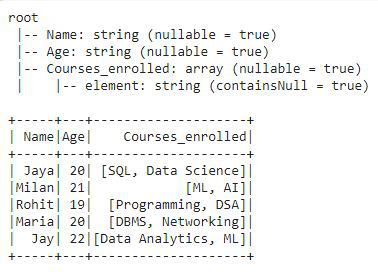In the schema of the Dataframe we can see that the first two columns have string-type data and the third column has array data. Now, we will split the array column into rows using explode().

## Python3

 `# using select function applying  ` `# explode on array column ` `df2 ``=` `df.select(df.Name,explode(df.Courses_enrolled)) ` ` `  `# printing the schema of the df2 ` `df2.printSchema() ` ` `  `# show df2 ` `df2.show()`

Output:

In this output, we can see that the array column is split into rows. The explode() function created a default column ‘col’ for the array column, each array element is converted into a row, and also the type of the column is changed to string, earlier its type was array as mentioned in the above df output.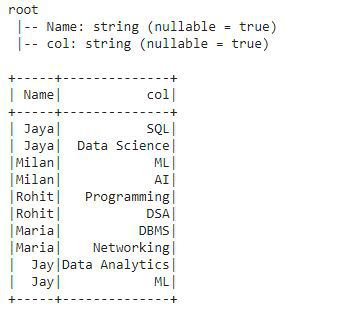## Types of explode() in PySpark

There are three ways to explode an array column:

• explode_outer()
• posexplode()
• posexplode_outer()

Let’s understand each of them with an example. For this, we will create a Dataframe that contains some null arrays also and will split the array column into rows using different types of explode.

## Python3

 `# creating the row data and giving array  ` `# values for dataframe along with null values ` `data ``=` `[(``'Jaya'``, ``'20'``, [``'SQL'``, ``'Data Science'``]), ` `        ``(``'Milan'``, ``'21'``, [``'ML'``, ``'AI'``]), ` `        ``(``'Rohit'``, ``'19'``, ``None``), ` `        ``(``'Maria'``, ``'20'``, [``'DBMS'``, ``'Networking'``]), ` `        ``(``'Jay'``, ``'22'``, ``None``)] ` ` `  `# column names for dataframe ` `columns ``=` `[``'Name'``, ``'Age'``, ``'Courses_enrolled'``] ` ` `  `# creating dataframe with createDataFrame() ` `df ``=` `spark.createDataFrame(data, columns) ` ` `  `# printing dataframe schema ` `df.printSchema() ` ` `  `# show dataframe ` `df.show() `

Output: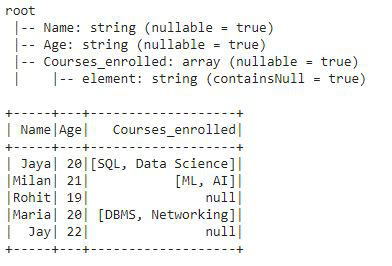### explode_outer() in PySpark

The explode_outer function splits the array column into a row for each element of the array element whether it contains a null value or not. Whereas the simple explode() ignores the null value present in the column.

## Python3

 `# now using select function applying ` `# explode_outer on array column ` `df4 ``=` `df.select(df.Name, explode_outer(df.Courses_enrolled)) ` ` `  `# printing the schema of the df4 ` `df4.printSchema() ` ` `  `# show df2 ` `df4.show() `

Output: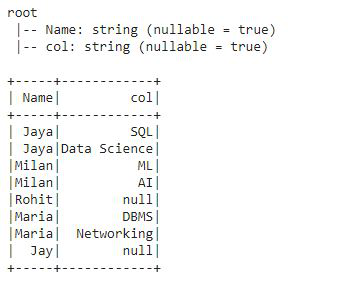As we have defined above that explode_outer() doesn’t ignore the null values of the array column. Clearly, we can see that the null values are also displayed as rows of Dataframe.

### posexplode() in PySpark

The posexplode() splits the array column into rows for each element in the array and also provides the position of the elements in the array.  It creates two columns “pos’ to carry the position of the array element and the ‘col’ to carry the particular array elements and ignores null values. Now, we will apply posexplode() on the array column ‘Courses_enrolled’.

## Python3

 `# using select function applying  ` `# explode on array column ` `df2 ``=` `df.select(df.Name, posexplode(df.Courses_enrolled)) ` ` `  `# printing the schema of the df2 ` `df2.printSchema() ` ` `  `# show df2 ` `df2.show() `

Output: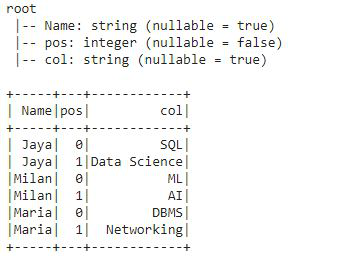As the posexplode() splits the arrays into rows and also provides the position of array elements and in this output, we have got the positions of array elements in the ‘pos’ column. And it ignored null values present in the array column.

### posexplode_outer() in PySpark

The posexplode_outer() splits the array column into rows for each element in the array and also provides the position of the elements in the array.  It creates two columns “pos’ to carry the position of the array element and the ‘col’ to carry the particular array elements whether it contains a null value also. That means posexplode_outer() has the functionality of both the explode_outer() and posexplode() functions. Let’s see this in an example.

Now, we will apply posexplode_outer() on the array column ‘Courses_enrolled’.

## Python3

 `# using select function applying  ` `# explode on array column ` `df2 ``=` `df.select(df.Name, posexplode_outer(df.Courses_enrolled)) ` ` `  `# printing the schema of the df2 ` `df2.printSchema() ` ` `  `# show df2 ` `df2.show() `

Output: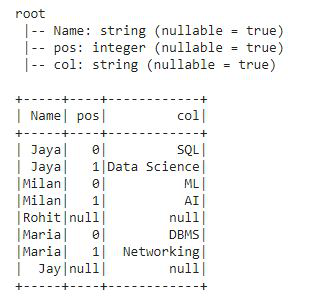As, posexplode_outer() provides functionalities of both the explode functions explode_outer() and posexplode(). In the output, clearly, we can see that we have got the rows and position values of all array elements including null values also in the ‘pos’ and ‘col’ columns.

Whether you're preparing for your first job interview or aiming to upskill in this ever-evolving tech landscape, GeeksforGeeks Courses are your key to success. We provide top-quality content at affordable prices, all geared towards accelerating your growth in a time-bound manner. Join the millions we've already empowered, and we're here to do the same for you. Don't miss out - check it out now!

Previous
Next